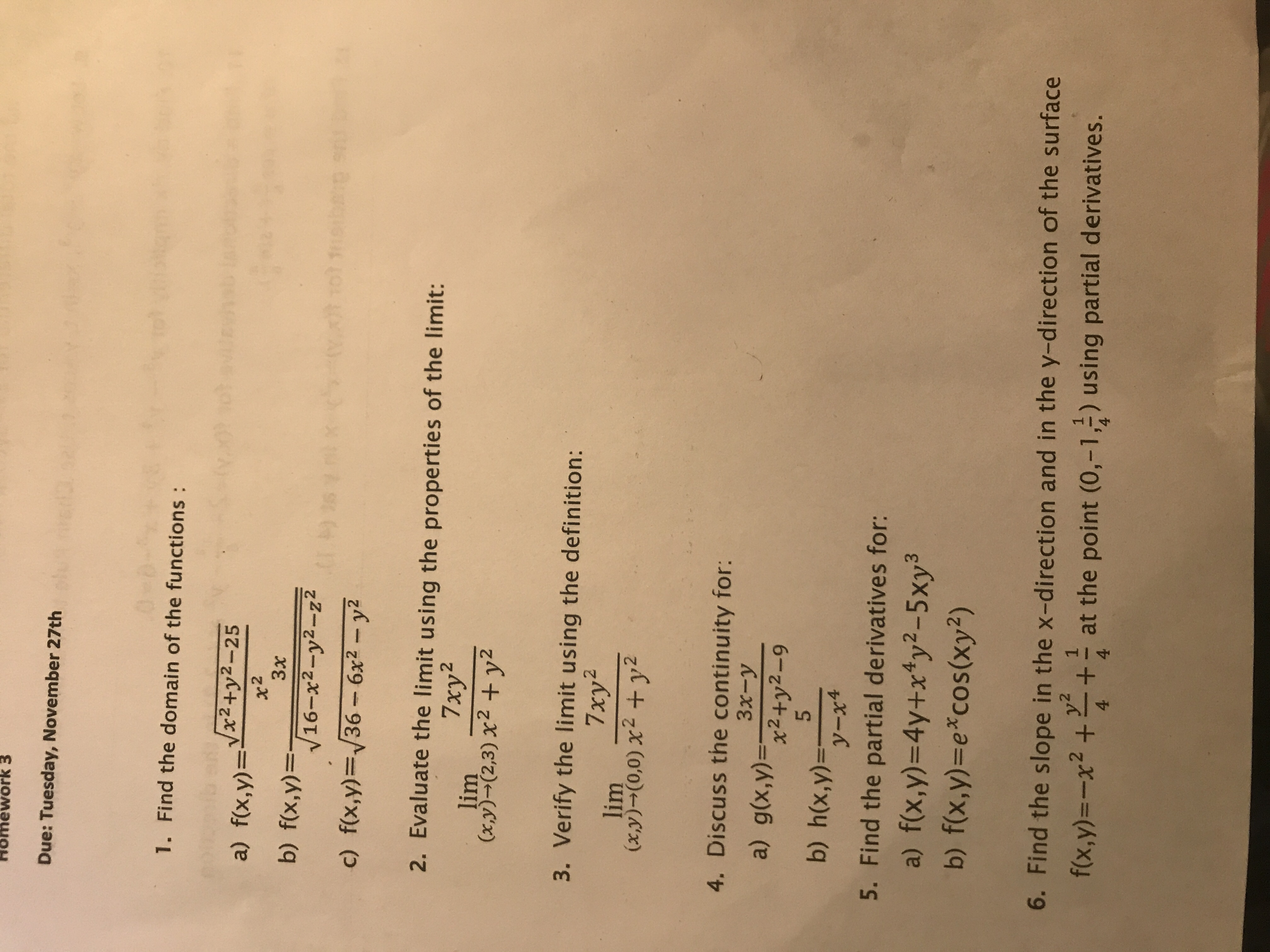# Homework 3 Due: Tuesday, November 27th 1. Find the domain of the functions a) fx,y)-32572-25 b) f(x,y)- c) f(x,y)-36- 6x2 y2 2. Evaluate the limit using the properties of the limit: 16-x2-y2-22 7xy2 lim (x,y)-(2,3) X2 + y2 3. Verify the limit using the definition: 7xy2 (x,y) (0,0)%2 + y2 4. Discuss the continuity for: 3x- x2+y2-9 5 b) h(x,y) 5. Find the partial derivatives for: 4,2 b) f(x,y)-e cos(xy2) 6. Find the slope in the x-direction and in the y-direction of the surface fix.y)-x2+ at the point (0,-1,2) using partial derivatives.

Questionhelp_outlineImage TranscriptioncloseHomework 3 Due: Tuesday, November 27th 1. Find the domain of the functions a) fx,y)-32572-25 b) f(x,y)- c) f(x,y)-36- 6x2 y2 2. Evaluate the limit using the properties of the limit: 16-x2-y2-22 7xy2 lim (x,y)-(2,3) X2 + y2 3. Verify the limit using the definition: 7xy2 (x,y) (0,0)%2 + y2 4. Discuss the continuity for: 3x- x2+y2-9 5 b) h(x,y) 5. Find the partial derivatives for: 4,2 b) f(x,y)-e cos(xy2) 6. Find the slope in the x-direction and in the y-direction of the surface fix.y)-x2+ at the point (0,-1,2) using partial derivatives. fullscreen

## Expert Answer

### Want to see this answer and more?

Experts are waiting 24/7 to provide step-by-step solutions in as fast as 30 minutes!*

*Response times vary by subject and question complexity. Median response time is 34 minutes and may be longer for new subjects.
Tagged in© 2021 bartleby. All Rights Reserved.